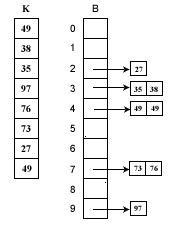# 桶排序

**桶排序的基本思想 **

** **假设有一组长度为N的待排关键字序列K[1….n]。首先将这个序列划分成M个的子区间(桶) 。然后基于某种映射函数 ，将待排序列的关键字k映射到第i个桶中(即桶数组B的下标 i) ，那么该关键字k就作为B[i]中的元素(每个桶B[i]都是一组大小为N/M的序列)。接着对每个桶B[i]中的所有元素进行比较排序(可以使用快 排)。然后依次枚举输出B….B[M]中的全部内容即是一个有序序列。

**[桶—关键字]映射函数 **

bindex=f(key)   其中，bindex 为桶数组B的下标(即第bindex个桶), k为待排序列的关键字。桶排序之所以能够高效，其关键在于这个映射函数，它必须做到：如果关键字k1<k2，那么f(k1)<=f(k2)。也就是说B(i)中的最小数据都要大于B(i-1)中最大数据。很显然，映射函数的确定与数据本身的特点有很大的关系，我们下面举个例子：**桶排序代价分析 **

(1) 循环计算每个关键字的桶映射函数，这个时间复杂度是O(N)。

(2) 利用先进的比较排序算法对每个桶内的所有数据进行排序，其时间复杂度为  ∑ O(Ni*logNi) 。其中Ni 为第i个桶的数据量。

(1) 映射函数f(k)能够将N个数据平均的分配到M个桶中，这样每个桶就有[N/M]个数据量。

(2) 尽量的增大桶的数量。极限情况下每个桶只能得到一个数据，这样就完全避开了桶内数据的”比较”排序操作。 当然，做到这一点很不容易，数据量巨大的情况下，f(k)函数会使得桶集合的数量巨大，空间浪费严重。这就是一个时间代价和空间代价的权衡问题了。

O(N)+O(M*(N/M)*log(N/M))=O(N+N*(logN-logM))=O(N+N*logN-N*logM)

```#include <stdio.h>

void swap(int *a, int *b)

{

int temp = *a;

*a = *b;

*b = temp;

}

int partition(int *array, int low, int high)

{

int middle = (low + high)/2;

for(int i=low,j=high; i<j; ) {

while(array[i] < array[middle]) ++i;

if(i < j && i != middle) {

swap(&array[i], &array[middle]);

middle = i;

}

while(array[j] > array[middle]) --j;

if(i < j && j != middle) {

swap(&array[j], &array[middle]);

middle = j;

}

}

return middle;

}

int quicksort(int *array, int low, int high)

{

int piovt_pos;

if(low < high) {

piovt_pos = partition(array, low, high);

quicksort(array, low, piovt_pos - 1);

quicksort(array, piovt_pos + 1, high);

}

}

struct barrel {

int a;

int count;

};

int main()

{

int data[] = {23, 12, 3, 54, 1, 98, 24, 34};

int min = data;

int max = data;

for(int i=1; i<sizeof(data)/sizeof(int); ++i) {

min = min < data[i] ? min : data[i];

max = max > data[i] ? max : data[i];

}

int num = (max - min + 1)/10 + 1;

barrel *pBarrel = new barrel[num];

for(int i=0; i<sizeof(data)/sizeof(int); ++i) {

int j = (pBarrel+((data[i]-min+1)/10))->count;

(pBarrel+((data[i]-min+1)/10))->a[j] = data[i];

(pBarrel+((data[i]-min+1)/10))->count++;

}

static int pos = 0;

for(int i=0; i<num; ++i) {

quicksort((pBarrel+i)->a, 0, (pBarrel+i)->count-1);

for(int j=0; j<(pBarrel+i)->count; ++j) {

data[pos++] = (pBarrel+i)->a[j];

}

}

for(int i=0; i<8; ++i) {

printf("%d ", data[i]);

}

return 0;

}```